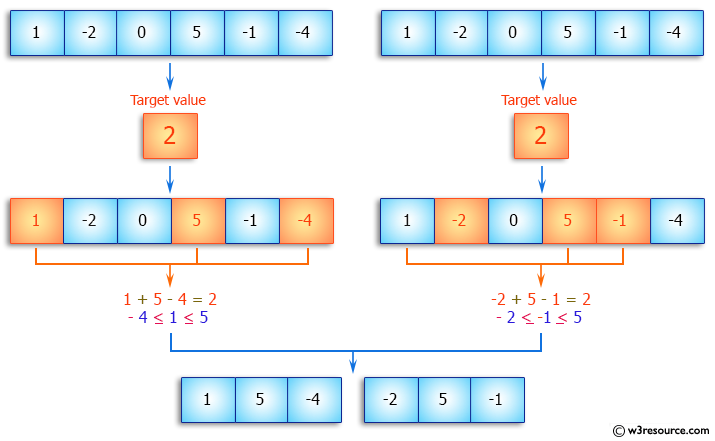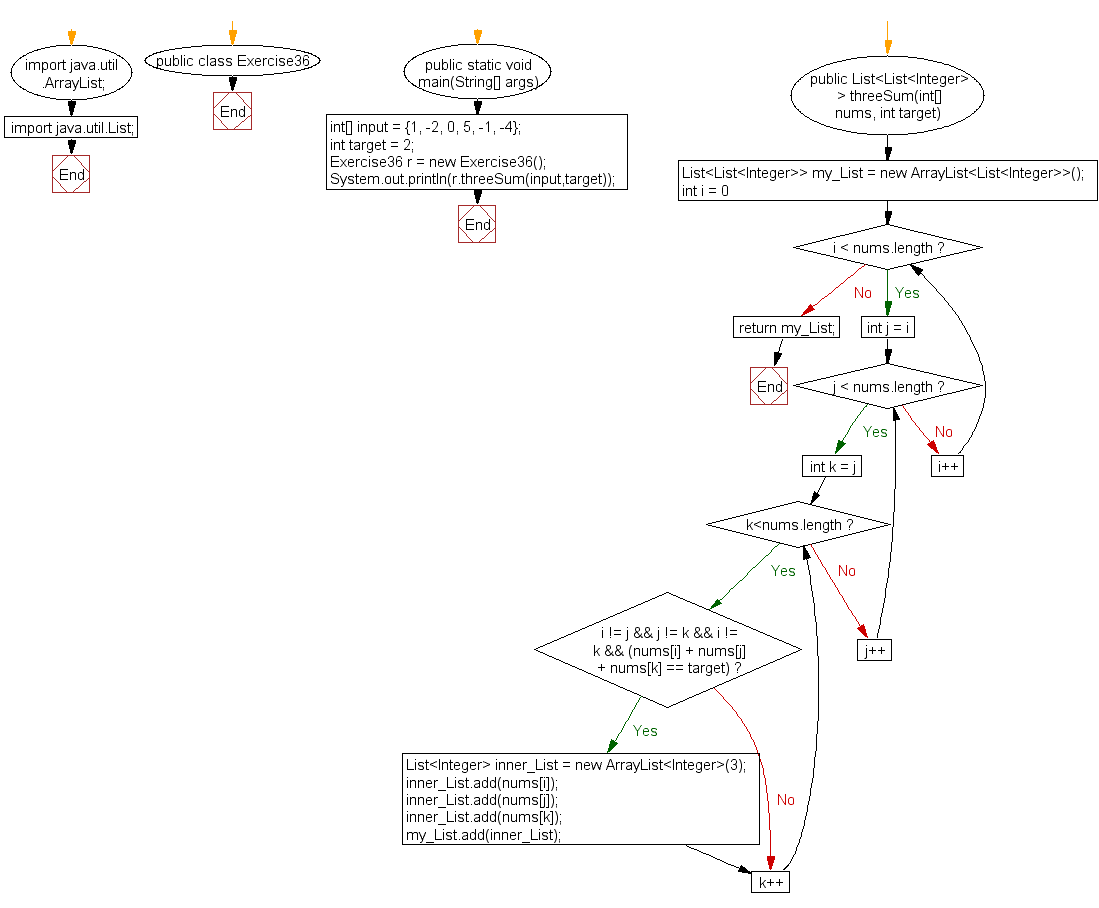﻿ Java: Finding all triplets whose sum equals a number# Java Array Exercises: Find all the unique triplets such that sum of all the three elements equal to a specified number

## Java Array: Exercise-36 with Solution

Write a Java program to find all the unique triplets such that sum of all the three elements [x, y, z (x ≤ y ≤ z)] equal to a specified number.
Sample array: [1, -2, 0, 5, -1, -4]
Target value: 2.

Pictorial Presentation:Sample Solution:

Java Code:

``````import java.util.ArrayList;
import java.util.List;
public class Exercise36 {
public static void main(String[] args) {
int[] input = {1, -2, 0, 5, -1, -4};
int target = 2;
Exercise36 r = new Exercise36();
System.out.println(r.threeSum(input,target));
}
public List<List<Integer>> threeSum(int[] nums, int target) {
List<List<Integer>> my_List = new ArrayList<List<Integer>>();

for(int i = 0; i < nums.length; i++){
for(int j = i; j < nums.length ;j++){
for(int k = j; k<nums.length;k++){
if ( i != j && j != k && i != k && (nums[i] + nums[j] + nums[k] == target)){
List<Integer> inner_List = new ArrayList<Integer>(3);
}
}
}
}
return my_List;
}
}
```
```

Sample Output:

```
[[1, 5, -4], [-2, 5, -1]]
```

Flowchart:Visualize Java code execution (Python Tutor):

Java Code Editor:

Improve this sample solution and post your code through Disqus

What is the difficulty level of this exercise?

Test your Programming skills with w3resource's quiz.

﻿

## Java: Tips of the Day

countOccurrences

Counts the occurrences of a value in an array.

Use Arrays.stream().filter().count() to count total number of values that equals the specified value.

```public static long countOccurrences(int[] numbers, int value) {
return Arrays.stream(numbers)
.filter(number -> number == value)
.count();
}
```

Ref: https://bit.ly/3kCAgLb